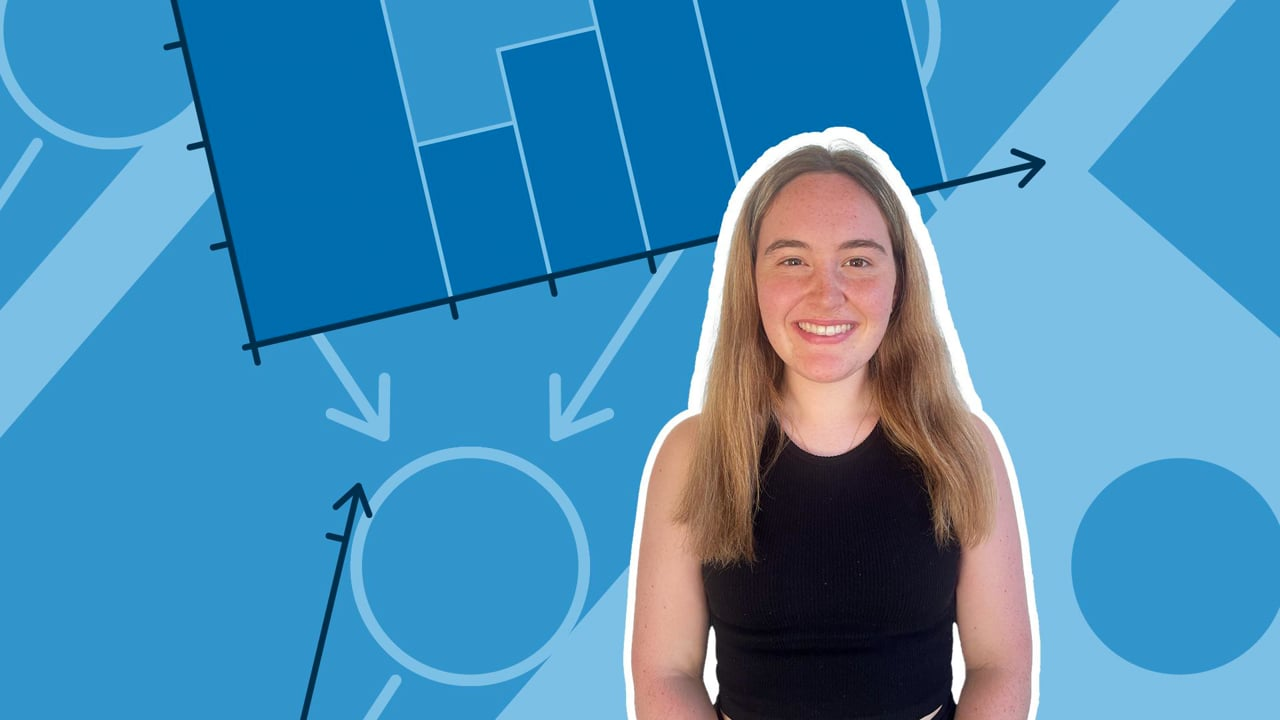Chapter OverviewMaths

Exam board

Pearson Edexcel

Number

Algebra

Graphs

Ratio proportion and rates of change

Shapes and area

Angles and geometry

Trigonometry

Probability

Statistics

Maths

# Mean, median, mode and range0%

Summary

# Mean, median, mode and range

## ​​In a nutshell

The mean, median, mode and range are used to compare and analyse data. They tell you information about a set of data such as the average or how big it is.

## What are the mean, median, mode and range?

Sometimes the mean, median, mode and range are referred to as averages but each one has their own definition.

### ​​Definitions

#### Mean

The average: the total of all the items added up $\div$ the total number of items.

#### Median

The middle value. The position can be found by using $\frac{n+1}{2}$ where n is the number of values.  (Note: The values MUST be in order from smallest to largest).

#### Mode

The most common value.

#### Range

The difference between the biggest and smallest

##### Example

What is the mean, median, mode and range of the following set of numbers?

$1,4,6,2,3,8,1,2,1,3$

Mean:

$\frac{\text{all the values added up}}{\text{the number of values}} = \frac{1+4+6+2+3+8+1+2+1+3}{10}=\frac{31}{10}=\underline{3.1}$

Median:

First, reorder the values.

$1,1,1,2,2,3,3,4,6,8$

Find the position of the median.

$\frac{n+1}{2} = \frac{11}{2} = 5.5$

Note: Since the position of the median is $5.5$, this means it sits in the middle of the fifth and sixth value. Add them together and divide by two to find the mean.

$(2+3)\div2=\underline{2.5}$

Mode:

The most common number in the sequence.

$\underline1$

Note: Sometimes there could be multiple modes if two values share the same frequency or no mode where all values are repeated the same number of times!

Range:

Take the smallest number away from the biggest number.

$8-1=\underline7$​​

​​​​

## Want to find out more? Check out these other lessons!

Mean, median, mode and range

FAQs

• Question: What is the range?

Answer: The range is the difference between the biggest and smallest values.

• Question: What is the mode?

Answer: The mode is the most common value.

• Question: What is the median?

Answer: The median is the middle value. It's position can be found by using (n+1) divided by two. (Note: the values MUST be in order from smallest to largest).

• Question: What is the mean?

Answer: The mean is the average: the total of all the items added up divided by the total number of items.

Theory

Exercises